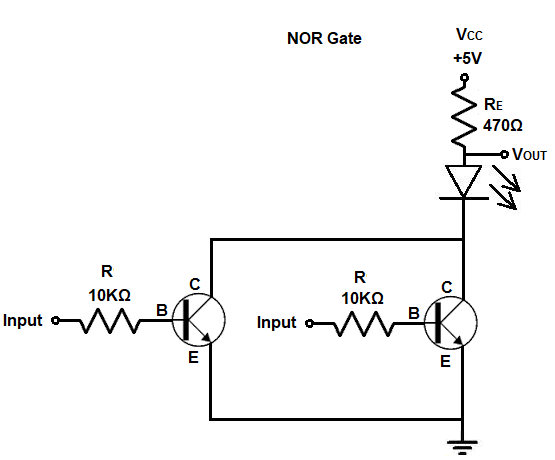﻿ How to Build a NOR Gate with Transistors ﻿# How to Build a NOR Gate with TransistorsIn this article, we go over how to build a NOR gate circuit with transistors.

Transistors are the building blocks of logic gates, such as NOT gates, OR gates, NOR gates, AND gates, and NAND gates, and gates are the building blocks of integrated circuits.

With transistors arranged in certain configurations, we can build the various gates that are used in electronics.

So from this article, you will not only learn how to build logic gates from discrete transistor components, but you learn more about how transistors operate in order to create gates. This teaches you more about the basic building blocks of electronic circuits, the transistor.

Any type of transistor can be used in order to create logic gates, including BJTs or FETs. However, in this article, we will use NPN BJTs in order to do it.

The 2N3904 transistor is a very common NPN BJT transistor which can act as a switch or an amplifier.

The NPN BJT transistor, just like a FET, has 3 terminals: these 3 terminals are the base, collector, and emitter.

The base functions as the turn-on switch. A small amount of current flowing through the base can allow a much larger current to flow from the collector to the emitter. The BJT functions as an electrical switch, in which if we feed a small current to the base of a transistor, it turns on a larger current from the collector to the emitter.

The components we need to build this circuit are shown below.

### Components Needed

• 2 2N3904 Transistors
• 2 10KΩ Resistor
• 470Ω Resistor
• LED

So with just a few components, we can construct a NOR gate circuit.

Know that a NOR gate circuit turns on a load if any of the inputs are a logic HIGH.

Below is the truth table for a NOR gate.

 NOR Gate Logic Inputs Output 0 0 1 0 1 0 1 0 0 1 1 0

So as you can see from the truth table, a NOR gate will have a HIGH output if all of its inputs is a logic LOW. Otherwise, it will have a logic HIGH state if any of the inputs are a logic HIGH.

The circuit we will build is shown below.So what makes this a NOR gate?

You can see that the transistors are in parallel with each other and that the load is connected to the collectors of the transistors. It is a NOR gate because it is the inverted output of an OR gate. The load turns on when both inputs are off. If either input turns on, the load turns off.

As stated before, transistors are the building blocks for ICs, because they form the logic gates which are used heavily in computers.

Arranging transistors in certain configurations allows us to build gates, which can then be used to form higher-level electronic devices such as registers, latches, etc.

Knowing how transistors can be used to create logic gates allows you to understand electronics at the lowest level, so that you can get the best understanding of it from the most basic fundamental level.

This, again, was done through NPN BJT transistors but it can also be done in a similar fashion with FETs such as MOSFETs.

So this is how to build a NOR gate with transistors.

Related Resources